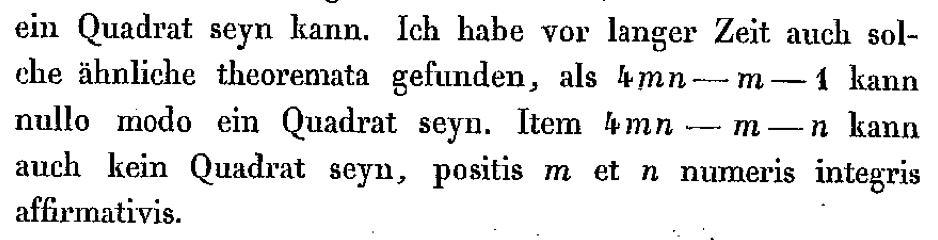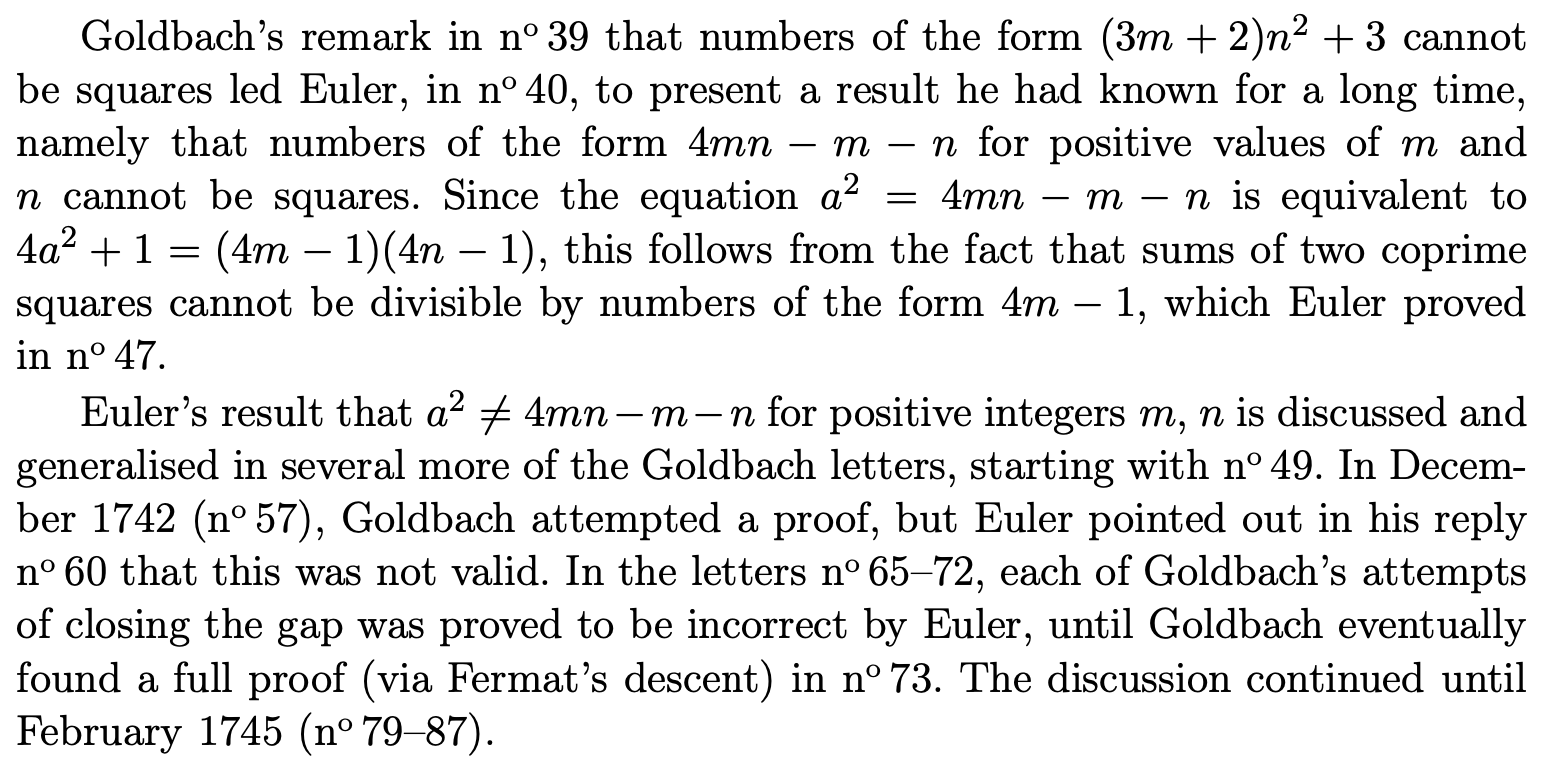# Origin and variations of problem on $4xy-x-y$ being square

One of the forms in which the Diophantine equation in question can be found in the literature is this:

Solve the equation $$\begin{eqnarray}z^{2} = 4xy-x-y \qquad \qquad (\ast)\end{eqnarray}$$ in positive integers $$x, y$$, and $$z$$.

There are some other variants of it here and there. It is usually given once the instructor has touched upon the Jacobi symbol.

My questions about the problem are the following two:

1. Do you happen to know where it was that the problem first appeared? If I understand correctly, the variation in which one is asked to establish that $$z^{2} = 4xyt^{u}-t^{v}-y$$ does not admit solutions in positive integers might have first appeared in a Russian compilation of problems of olympiad caliber...

2. What other notable variations of (*) do you know?

• artofproblemsolving.com/community/… – individ Jul 5 '20 at 4:24
• Looking at the two answers, I wonder (1) how accessible the Euler--Goldbach correspondence was in London around 1900 and (2) whether Euler or Goldbach considered the triangular number result that Crofton included. – Brian Hopkins Jul 9 '20 at 3:04
• @BrianHopkins Euler's writings and correspondence were published separately. – individ Jul 9 '20 at 4:21
• @individ Yes, Fuss published some of the correspondence in 1845, including the 9 September 1741 letter that Carlo detailed (now indexed as OO754). I still wonder how accessible that was to Crofton. – Brian Hopkins Jul 9 '20 at 21:35
• I don't know why, but the "here and there" phrase with "here" pointing somewhere really plays with my head. I'm emotionally unable to concentrate on the real content of the post. – Mohsen Shahriari Jul 11 '20 at 12:36

I found an 1899 reference in Google Books: Mathematical Questions and Solutions from the "Educational Times" edited by D. Biddle, vol. 70, Hodgson, London, 1899. On page 73:

1. (Professor Crofton, F.R.S.) Show that $$4mn-m-n$$ cannot be (1) a square; nor (2) a triangular number.

Brief solutions by H. W. Curjel and Allan Cunningham are given. It looks like the question was posed in the February 1, 1898 issue of The Educational Times and Journal of the College of Preceptors (vol. 51, page 87).

Here are the solutions, which could help with making variant problems.

Solutions (1) by H. W. Curjel, M.A.; (2) by Lt.-Col. Allan Cunningham, R.E.

(1) If $$4mn - m - n = x^2 \quad \text{or} \quad \frac{1}{2}\{y(y+1)\},$$ $$(4m-1)(4n-1) = 4x^2 + 1 \quad \text{or} \quad \frac{1}{2}\{(2y+1)^2+1\},$$ which is impossible, since all the prime factors of $$X^2+1$$ other than 2 are of the form $$4N+1$$.

(2) Here $$4mn - m - n = \frac{1}{4}(4m-1)(4n-1) - \frac{1}{4}.$$ (i) Let this $$= z^2$$ (if possible) [I think that should be $$x^2$$]. Then $$(4m-1)(4n-1) = 1+(2x)^2,$$ which gives a sum of squares $$\{1^2+(2x)^2\} =$$ product of two numbers of form $$(4m-1)$$, which is impossible.

(ii) Let $$4mn-m-n = \frac{1}{2}(x^2+x)$$, a triangular number (if possible). Therefore $$(4m-1)(4n-1) = 2x^2 + 2x + 1 = (x+1)^2 + x^2,$$ a sum of squares, which is impossible, as before.

• No. The first mention of this equation was in the works of Euler. The given articles are a rewritten result of it. artofproblemsolving.com/community/c3046h1049059__ – individ Jul 8 '20 at 4:31
• A triangular number can't be? Write the equation as you imagine it. Its shape can be different. – individ Jul 8 '20 at 4:40
• @individ It would be great to have a specific citation for where Euler discussed this. Regarding your question about the triangular number result, I've included the 1899 published solutions. – Brian Hopkins Jul 8 '20 at 15:13
• @individ --- great find, a 1741 letter by Euler to Goldbach indeed discusses this result! – Carlo Beenakker Jul 8 '20 at 15:48

A twentieth century source for this problem is the Canadian entry to the 1984 International Mathematics Olympiad. The original handwritten document of the full problem set can be downloaded here.

For variations, in addition to $$z^{2} = 4xyt^{u}-t^{v}-y$$ [link], there is: $$x,y,m,n,k$$ are natural; prove that $$4kxy-1 \nmid x^m+y^n$$ [link]

Addendum: following up on individ's pointer, here is a two-and-a-half century older attribution to Euler, in a letter to Goldbach dated 9 September 1741, see page 107 of this source.I have also found similar theorems a long time ago, such as that $$4mn-m-1$$ can never be a square. Likewise $$4mn-m-n$$ can never be a square, assuming that $$m$$ and $$n$$ are integer numbers.

A discussion of this result of Euler follows on page 45 of this source.• Thanks for digging this up, Carlo. And that's a wonderful link! – Brian Hopkins Jul 8 '20 at 16:15
• @BrianHopkins, Carlo Beenakker: Thank you very much for taking the time to leave your replies... I did not know (or recall) that the "original" problem could be found in the Goldbach-Euler Briefwechsel. – José Hdz. Stgo. Jul 8 '20 at 22:21